# CBSE Class 12 Physics Chapter 4 Revision Notes

Chapter 4: Moving Charges and Magnetism

• Magnetic field is the area around a magnet or a current-carrying conductor in which its magnetic influence can be felt. Tesla is the SI unit for it (T).
• Oersted demonstrated that the current-carrying conductor generates a magnetic field around it in an experiment.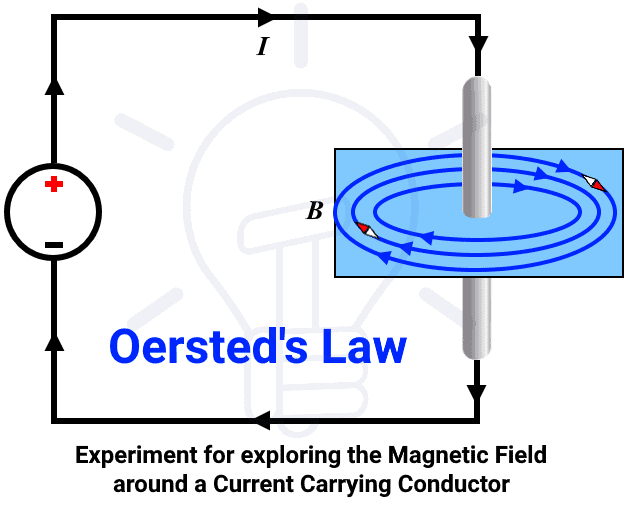Source

• The Law of Biot-Savart: The magnetic field due to a small current-carrying element dl at any nearby point P is given by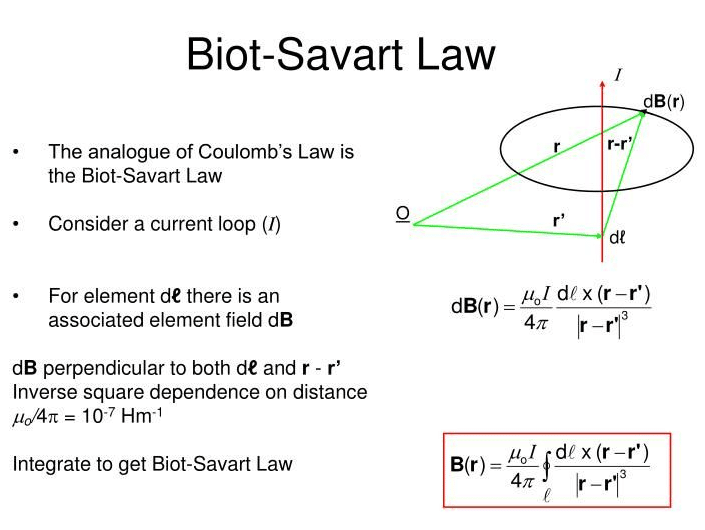Source

• A magnetic field in the center of a circular current-carrying conductor or coil.

• Magnetic field in the semi-circular current-carrying conductor’s center.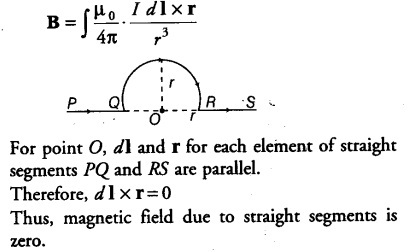Source

• Magnetic field in the center of a circular current-carrying conductor arc with an angle of 0 at its center.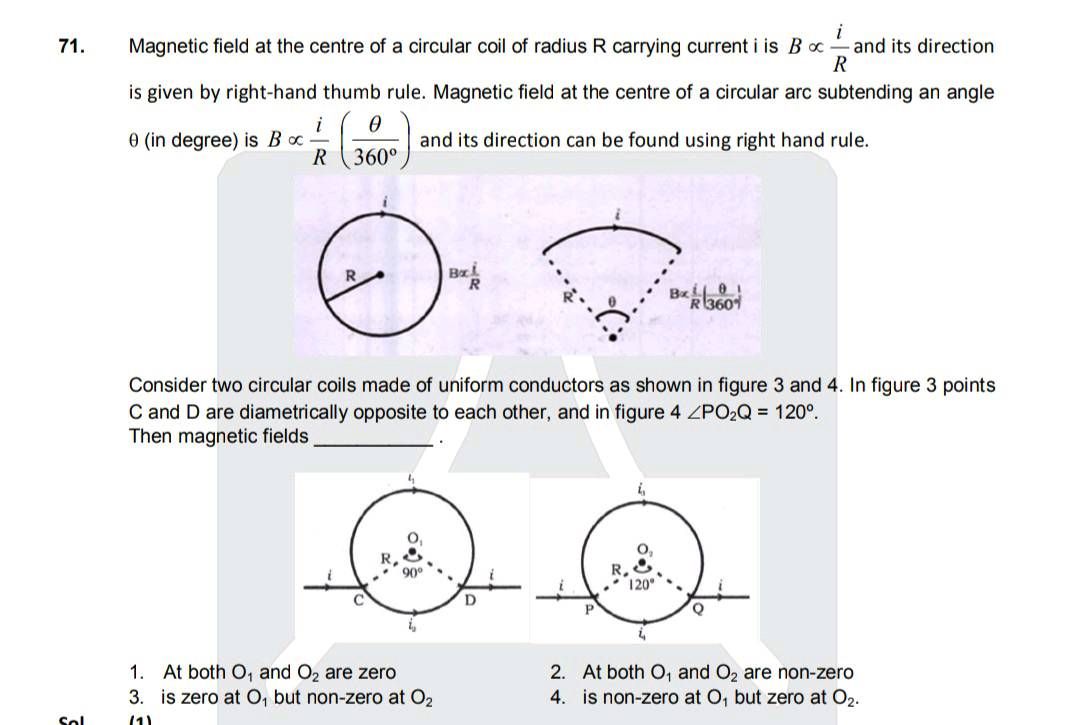Source

• Any point on the axis of a circular current-carrying conductor has a magnetic field.

## Ampere’s Circuital Law

• Any closed loop’s line integral of the magnetic field B is equal to 0 times the total current I threading through the loop, i.e.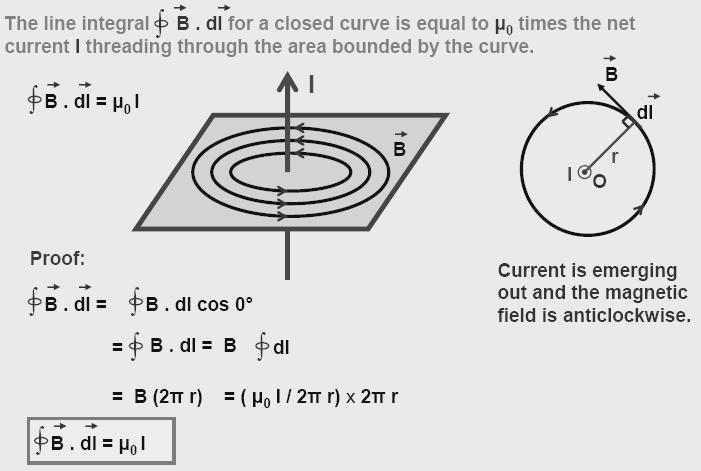Source

• Maxwell popularized the term “displacement current.”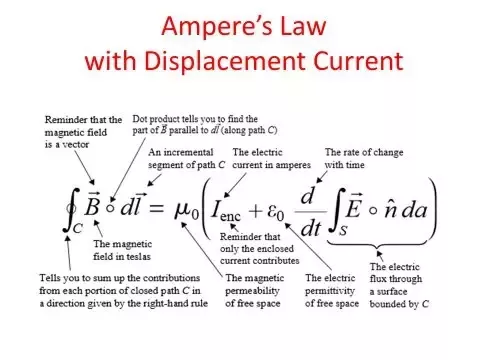Source

]]>